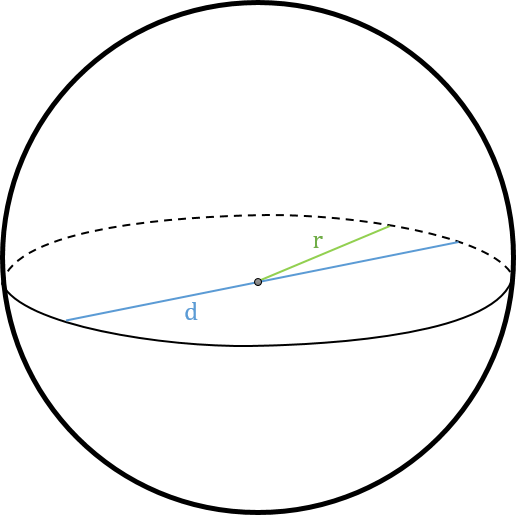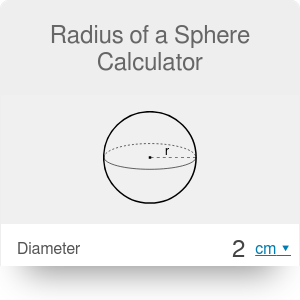Diameter
in
Surface area
in²
Volume
cu in
Surface to volume ratio
1/
in
in

# Radius of a Sphere Calculator

By Dominik Czernia, PhD candidate

Our radius of a sphere calculator is a perfect tool that can estimate every parameter of a sphere from just one another quantity. However, it is mainly designed to support the computation of sphere radius. Try this calculator now by entering one of the chosen parameters into the appropriate field or read on and learn how to find the radius of a sphere. In the following text, we have also presented four different radius of a sphere formulas.

A sphere is a perfectly round geometrical object in 3D space. The points on its surface are equally distant from the center of a sphere. It is an analogue to a circle in 2D space. Radius of a sphere calculator uses five variables that can completely describe any sphere:A sphere is a special object because it has the lowest surface to volume ratio among all other closed surfaces with a given volume. Also here we can find the analogy to the circle, which encloses the largest area with a given perimeter. This radius of a sphere calculator, as the name suggests, contains information dedicated mostly to the radius of a sphere. For more general information about spheres check out our sphere calc!

There is an object called hemisphere that you can construct from any sphere you want. You just need to divide a sphere into two equal parts. Description of a hemisphere is a little bit more complicated compared to the full sphere, but it is possible. If you want to learn more about that kind of objects, check out area and volume of a hemisphere calculators!

## What's the radius of a sphere formula?

How to find the radius of a sphere? Actually, there are many various answers to that question because it depends on what we know about a specific sphere. Below, we have provided an exhaustive set of a radius of a sphere formulas:

1. Given diameter: `r = d / 2`,
2. Given area: `r = √[A / (4 * π)]`,
3. Given volume: `r = ³√[3 * V / (4 * π)]`,
4. Given surface to volume ratio: `r = 3 / (A/V)`.

Our radius of a sphere calculator uses all of the above equations simultaneously, so you need to enter just one chosen quantity. Moreover, you can freely change the units (SI and imperial units). Check out our length conversion to learn how to switch between different units of length!

Derivation of the above radius of a sphere formulas is, in fact, straightforward. You need to make some algebraic transformations using the following basic equations:

1. Diameter of a sphere: `d = 2 * r`,
2. Surface area of a sphere: `A = 4 * π * r²`,
3. Volume of a sphere: `V = 4/3 * π * r³`,
4. Surface to volume ratio of a sphere: `A / V = 3 / r`.

## How to find the radius of a sphere?

This question seems very easy at first. You only have to find the center of the sphere and measure the distance to any point on its surface. However, how can you find this center in real, physical sphere, especially when it's closed? Let's look on a two of our suggestions:

1. Find the diameter. You can use two parallel planes and put the sphere between them. The distance between those plates equals the diameter of a sphere.
2. Find the volume. Prepare a graduated cylindrical container filled with water. Put a sphere into this cylinder, then bring out the sphere and measure the volume of displaced water (you can use our volume of a cylinder calculator to do so). If you want to see how objects behave in liquids, try our buoyancy calculator now!

We didn't write about finding the surface area of sphere first, because it is much harder to do it (compared to finding the radius).

It is worth mentioning that spheres can often simplify various problems in physics. That's why they are widely used in this field, e.g. to model spherical capacitors or atoms of a gas.

Dominik Czernia, PhD candidate

## Get the widget!

Radius of a Sphere Calculator can be embedded on your website to enrich the content you wrote and make it easier for your visitors to understand your message.

It is free, awesome and will keep people coming back!